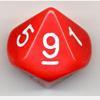# Search by Topic

Filter by: Content type:
Age range:
Challenge level:

### There are 9 results

Broad Topics > Numbers and the Number System > Rounding### Reasoned Rounding

##### Age 7 to 14 Challenge Level:

Four strategy dice games to consolidate pupils' understanding of rounding.### Round the Dice Decimals 1

##### Age 7 to 11 Challenge Level:

Use two dice to generate two numbers with one decimal place. What happens when you round these numbers to the nearest whole number?### Round the Two Dice

##### Age 5 to 7 Challenge Level:

This activity focuses on rounding to the nearest 10.### Round the Dice Decimals 2

##### Age 7 to 11 Challenge Level:

What happens when you round these numbers to the nearest whole number?### Round the Four Dice

##### Age 7 to 11 Challenge Level:

This activity involves rounding four-digit numbers to the nearest thousand.### Rough Rectangle

##### Age 11 to 14 Short Challenge Level:

What is the smallest possible area that this rectangle could have?### Round the Three Dice

##### Age 7 to 11 Challenge Level:

What happens when you round these three-digit numbers to the nearest 100?### Archimedes and Numerical Roots

##### Age 14 to 16 Challenge Level:

The problem is how did Archimedes calculate the lengths of the sides of the polygons which needed him to be able to calculate square roots?### Route to Root

##### Age 16 to 18 Challenge Level:

A sequence of numbers x1, x2, x3, ... starts with x1 = 2, and, if you know any term xn, you can find the next term xn+1 using the formula: xn+1 = (xn + 3/xn)/2 . Calculate the first six terms of this. . . .